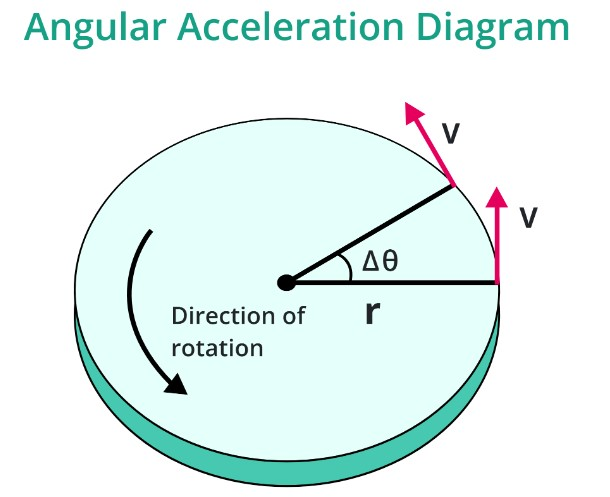# Angular Acceleration Calculator

To calculate angular acceleration, input the values of torque and moment of inertia in the angular acceleration calculator.

Formula:
α = 𝜏/I
sec

Give Us Feedback

## Angular Acceleration Calculator

This angular acceleration calculator helps to find the acceleration of a rotating body if the moment of inertia and total torque are given.

## What is Angular Acceleration?

According to Wikipedia:

“Angular acceleration refers to the time rate of change of angular velocity.”

In simple words,

“It is the rotation of a rigid body about a fixed center point.”## Angular acceleration formula

The equation used for the calculation of angular acceleration is:

α = T/I

Where,

• α represents angular acceleration,
• T represents torque, and
• I is moment of inertia.

The formula used in this calculator is related to tangential acceleration as:

αt = αr

Where αt is tangential acceleration.

## How to find angular acceleration?

Example

Calculate angular acceleration if the body rotates at 30 radians per second and the moment of inertia is 10 kgm2.

Solution:

Step 1: Identify the values.

I = 10 kgm2

Step 2: Put the values into the formula.

α = T/I

α = 30/10